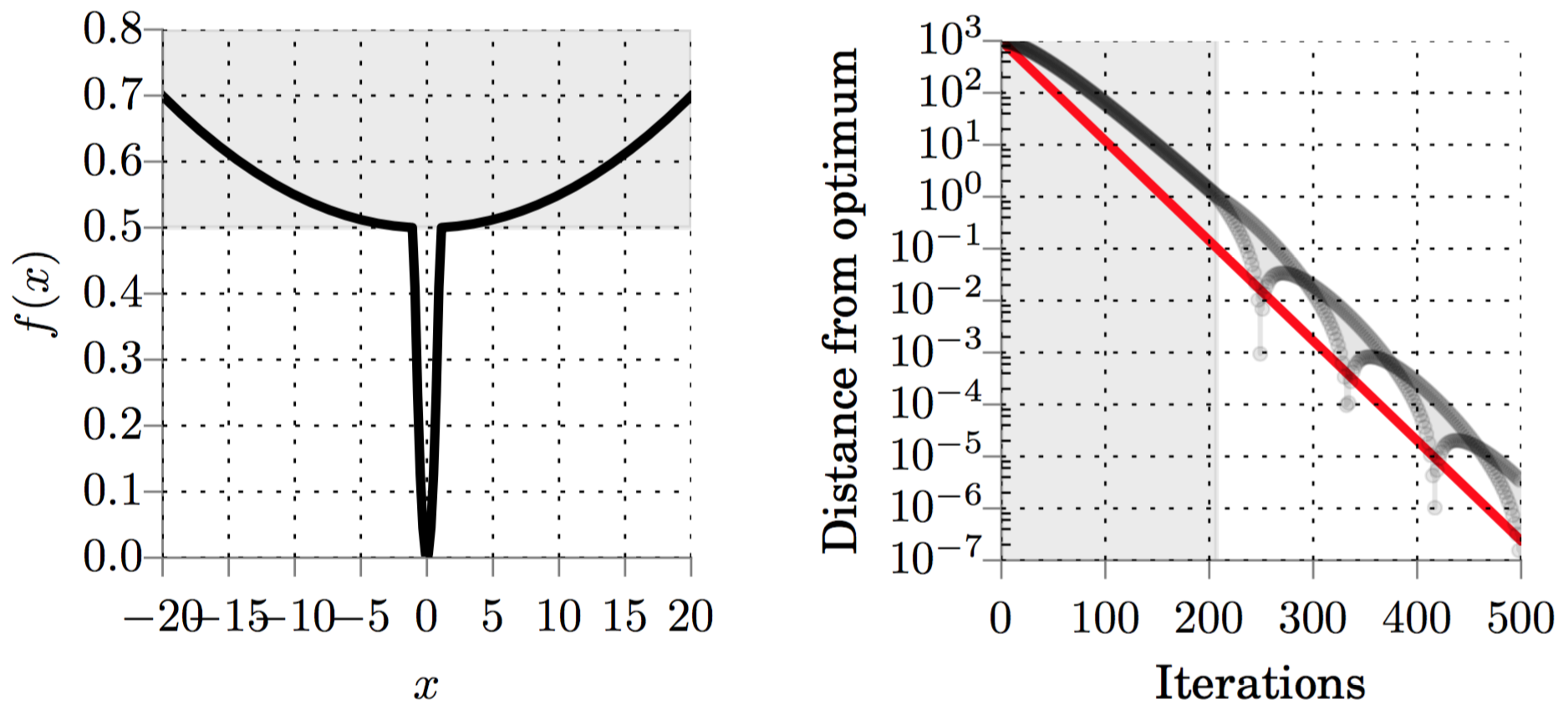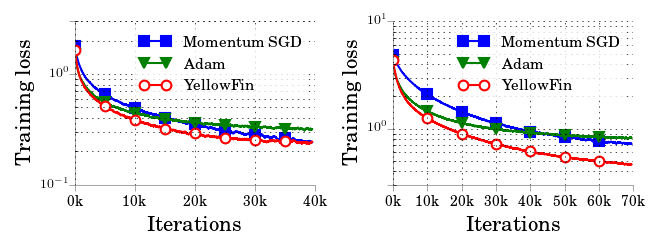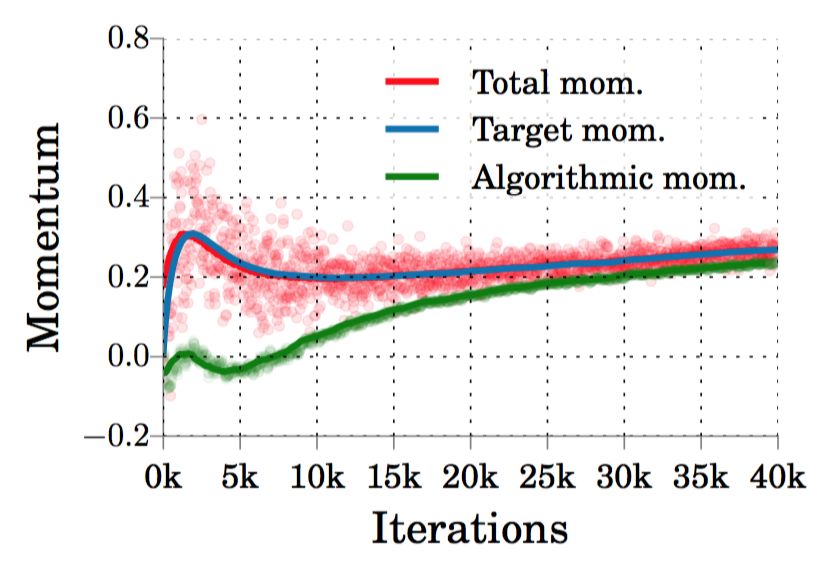# YellowFin and the Art of Momentum Tuning

by Jian Zhang, Ioannis Mitliagkas and Chris Ré.

TLDR; Hand-tuned momentum SGD is competitive with state-of-the-art adaptive methods, like Adam. We introduce YellowFin, an automatic tuner for the hyperparameters of momentum SGD. YellowFin trains large ResNets and LSTMs in fewer iterations than the state-of-the-art. It performs even better in asynchronous settings via an on-the-fly momentum adaptation scheme that uses a novel momentum measurement component along with a negative-feedback loop mechanism.(Figure) Comparing YellowFin to Adam on training a ResNet on CIFAR100 (left) synchronously; (right) asynchronously, using 16 workers.

Hyperparameter tuning is one of the most painful part of any deep learning pipeline. The literature is full of amazing results that seem to be powered by black magic: they work because the authors/engineers/grunts spent an obscene amount of time exploring the hyperparameter space. Finding a working configuration can be a very frustrating affair.Methods like Adam and RMSProp tune learning rates for individual variables and make life easier.

Our experimental results show that those adaptive methods do not perform better than carefully tuned, good ol' momentum SGD.

This understanding is supported by recent theoretical results, which also suggest adaptive methods can suffer from bad generalization. The hypothesis is that variable-level adaptation can lead to completely different minima. Here we point out another important, overlooked factor: momentum.

Momentum tuning is critical for efficiently training deep learning models.

Classic convex results, and recent papers study momentum and emphasize its importance. Then, there are the dynamics of asynchrony: our recent paper shows that training asynchronously introduces momentum-like dynamics in the gradient decent update. Those added dynamics make momentum tuning even more important. Sometimes even negative momentum values can be optimal!

Despite these good reasons, the state of the art does not tune monentum!

The majority of deep learning literature sticks to the standard 0.9, leaving significant performance improvements on the table. It is no accident that the most successful GAN papers hand-tune the momentum parameter to a small positive or zero value.

### YellowFin's momentum

We revisit SGD with Polyak's momentum, study some of its robustness properties and extract the design principles for a tuner, YellowFin. YellowFin automatically tunes a single learning rate and momentum value for SGD.The rest of the post focuses on:

• Robustness: Momentum is robust in curvature variation. Empirically, this can yield a constant rate of convergence for some non-convex objectives. This observation powers YellowFin's momentum tuning.
• On-the-fly asynchrony compensation: We can measure the total momentum in a system, including any asynchrony-induced momentum. We use a simple negative-feedback loop to control the algorithmic momentum value.
• Flying fish: Experimental results on synchronous and asynchronous settings show that YellowFin requires fewer iterations to train ResNets and large LSTMs compared to Adam.

To try our tuner, get your fins on here for Tensorflow and here for PyTorch. For full technical details, please refer to our paper YellowFin and the Art of Momentum Tuning on arXiv.

## Robustness properties of momentum

Let us focus, for now, on quadratic objectives. At the heart of YellowFin, there is a very simple technical nugget.

Lemma. We call a specific family of hyperparameter configurations the robust region. Roughly speaking, this happens when momentum, μ, is high enough and the learning rate is in a specific range, which grows with μ.

In the robust region, the momentum algorithm converges at a constant rate equal to the root of momentum, and independent of the learning rate.

Caveat: It should be noted that this result on the spectral radius does not necessarily imply a convergence guarantee for non-quadratic objectives. In fact, there are counter examples within the class of strongly convex functions, where Polyak's momentum does not converge.

Still, we observe in practice that proper momentum tuning on some non-convex toy models (left) can yield the rate predicted by theory (right):(Figure) Constant convergence rate on a toy non-convex objective.

We validate this on on real models, like the LSTM in the following figure. We observe that for large values of momentum, most variables (grey lines) follow the root μ convergence rate (red line).(Figure) Constant convergence rate when training a real model (LSTM).

This observation informs YellowFin's design principles.

## YellowFin's design and results

Design principle 1: Stay in the robust region.

We tune the momentum value to keep all variables in the robust region. On a quadratic approximation, this guarantees convergence of all model variables at a common rate, though it empirically extends to certain non-convex objectives.

To tune momentum, YellowFin keeps a running estimate of curvatures along the way. This estimate doesn't need to be accurate. Using a very rough measurement from noisy gradients can betray sufficient information about the generalized condition number. This design principle suggests a lower bound on the value of momentum.

Design principle 2: Optimize hyperparameters at each step to minimize a local quadratic approximation.

Please refer to our paper YellowFin and the Art of Momentum Tuning for full implementation details.

#### Results

Our experiments show that YellowFin, without tuning, needs fewer iterations than tuned Adam and tuned momentum SGD to train ResNets and LSTMs.(Figure) Training loss for tuned momentum SGD, tuned Adam, and YellowFin on (left) 110-layer ResNet for CIFAR10 and (right) 164-layer ResNet for CIFAR100.(Figure) LSTM test metrics for tuned momentum SGD, tuned Adam, and YellowFin on (left) word-level language modeling; (middle) character-level language modeling; (right) constituency parsing.## Asynchronous dynamics and Closed-loop YellowFin

Asynchrony induces momentum. This result means that when we run asynchronously, the total momentum present in the system is strictly more than the algorithmic momentum value.

In our paper we demonstrate for the first time that it is possible to measure total mometum. The next figure shows that our measurement matches the algorithmic value exactly when training synchronously (left). On asynchronous systems, however, the measured total momentum is strictly more that the algorithmic value (right).(Figure) Measured total momentum (left) matches the algorithmic value in the synchronous case; (right) is higher than the algorithmic value, when using 16 asynchronous workers.

This momentum excess can be bad for statistical efficieny. Our ability to measure total momentum allows us to compensate for asynchrony on the fly. Specifically, we use a negative feedback loop to make sure the measured total momentum tracks the target momentum decided by YellowFin.(Figure) Closing the momentum loop on 16 asynchronous workers: the negative feedback loop uses the total momentum measurement to reduce the algorithm momentum value. The end result is that total momentum closely follows the target value.

### Results

Closing the momentum loop results in less algorithmic momentum, sometimes even negative! Here we see that this adaptation is very beneficial in an asynchronous setting. (Open-loop) YellowFin already performs better that Adam, mostly due to its ability to reach lower losses. However, when we close the loop, the result is about 2x faster (and almost 3x faster to reach Adam's lowest losses).(Figure) Closing the momentum loop on 16 asynchronous workers: the negative feedback loop uses the total momentum measurement to reduce the algorithm momentum value. The end result is that total momentum closely follows the target value.## Conclusion

YellowFin is an automatic tuner for momentum SGD that is competitive with state-of-the-art adaptive methods that use individual learning rates for each variable.

In asynchronous settings, it uses a novel closed-loop design which significantly reduces the iteration overhead.

U.S. wild-caught Pacific yellowfin tuna is a smart seafood choice because it is sustainably managed and responsibly harvested under U.S. regulations. [fishwatch.gov]

## What's next

• YellowFin assumes some measurement oracles for quantities like curvature variation and gradient variance to tune its hyperparameters. For our first implementation we used some simple approximations based solely on gradients that give us good results. It would be, nonetheless, interesting to study different implementations for those oracles, e.g. using a backprop-based estimate for the Hessian diagonal, or smart line-searches to estimate the distance from a local minimum.
• Computational optimizations: so far we focused on showing that it is possible to engineer a smart momentum tuner for plain momentum SGD that is competitive with the state of the art in terms of statistical efficiency (the number of iterations to solution). Next we will be working on minimizing the computational overhead of our tuner.
• In our paper we show that hand-tuning Adam's momentum can improve its asynchronous performance. We believe that designing and a implementing our momentum measurement and negative feedback loop for other methods can make them better under asynchrony.